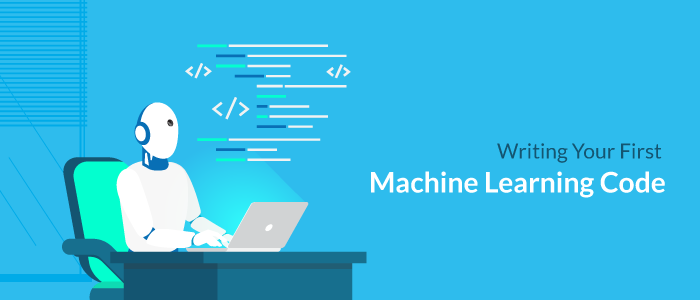## Writing your first machine learning codeRated 4.0/5 based on 20 customer reviews
Normal Mode Dark Mode

# Writing your first machine learning code

Ruslan Bragin
Tutorials
04th Oct, 2017The whole world is buzzing with artificial intelligence these days. Some people predict that it might change the way the world works into the future. For developers, it presents us with a fresh opportunity to be a part of a new paradigm shift.

I started learning AI about two months back and it has been a long road since. There are lots of developments happening in AI every day. From bots that develop their own language to an AI that can beat professional players in DOTA. From self-driving cars to computers that are better at diagnosing patients than experienced doctors. There is a lot of ground to cover.

Before we go further, we must understand that there are a lot of disciplines in AI. Some of them are easier to get into than others. Obviously, your first AI program cannot be to make a self-driving car. For beginners, it is best to start with a branch of machine learning  called supervised learning.

What is supervised learning?

Easily put, supervised learning means you give a bunch of data to a computer program and it uses mathematical models to draw inferences on that data. This type of learning is used for very simple regression and classification problems, but are really handy in solving many real-world problems.

Let’s start

Consider this very simple data set that I’ve prepared artificially just for the purpose of this example. This dataset follows three numbers X, Y, and Z. There exists a relationship between X, Y, and Z that we don’t know yet. Our goal in writing this program is to find this relationship. This data also contains some noise that is usually present in most real data sets.

82.95761557036997,15.49770283330364,53.746988734062285
41.831058370415896,74.6908398387234,76.91731716843984
0.45109458673243674,15.880369177717512,12.400022057356612
65.84526760369872,29.757778929447664,55.432668761221926
38.02804990326463,94.94571562617034,90.18671003364872
… and 95 more simple pairs like this.

For us to understand much easier, let’s put the equation in mathematical terms-
Z = a*X + b*Y

Our goal is to find a and b so that we can use any other pairs of X and Y to calculate Z. Such kinds of problems are solved by a mathematical technique called linear regression.

It is one of the most simple applications of Machine Learning and easiest to get into. Before we get into the more difficult aspects of machine learning, later on, it is important to understand that this exercise is very helpful to build up confidence in this field.

After all, bigger battles are won with the confidence of smaller victories.

Applications of linear regression

These values of X, Y and Z can represent anything. They can be any three variables that are related by a linear equation. For example, if you remember high school kinematics, the velocity of any given object at a given time when it starts from an initial velocity under the influence of gravity is given by the equation v(t) = u + At where A is a constant gravitational acceleration that we don’t know.

If we get a lot of experimental values for u, v and t, running a simple multivariate linear regression over these values can give us the coefficient values of u and t. We will find after our analysis that the coefficient of u will come out to be 1. If our data is to be accurate, we will get the value of A as 9.8 meters per second squared.

While this is just one area I have used for illustration, it is important to understand that linear relationships occur everywhere in nature.

Getting into the code

We will use Javascript to write this simple regression program by using an npm module called smr.  To set up the project, you must have nodejs installed. We could have used python as well, but installing and working with Scipy is not in our scope for now. We’ll gradually go there with time. For the time being,

Start by creating an empty directory for your project
Install smr by using npm install smr.
Create a new file called index.js and put in the code.

The code is as follows:

```var smr = require('smr'); var regression = new smr.Regression({ numX: 2, numY: 1 })```

```//read all the data by creating a read stream var lineReader = require('readline').createInterface({   input: require('fs').createReadStream('data.txt') });```

```//read it line by line and fit it into the regression object lineReader.on('line', function (line) {     line = line.split(',')     regression.push({x:[line,line], y:[line]})     t = regression.calculateCoefficients()     console.log(t) });```

You can get the dataset from this GitHub repo that I’ve created for this code at the end of this article.

Running the code

We run the code by using node index.js on the same directory as the code. Before we do that, let’s remember the data is in the form z = a*X + b*Y

The code fits the data into the regression object and prints coefficient values of a and b. When we run the code, we begin to see this output.

[ [ 0.5007233183107977 ], [ 0.7496919531492985 ] ]
[ [ 0.5007720353300695 ], [ 0.749708917362593 ] ]
[ [ 0.500762395739748 ], [ 0.7497046748655358 ] ]
[ [ 0.5007149604193888 ], [ 0.7496660120512422 ] ]
[ [ 0.5009035966316537 ], [ 0.7495249465185432 ] ]

The value of a begins to converge at 0.50 and the value of y begins to converge at 0.75. This is remarkable, as when you see the repo there is a quick python script that is used to prepare the data. The value of a and b that I had chosen were indeed 0.50 and 0.75.

That means our model is good and we have successfully created our first machine learning program.### Ruslan Bragin

Author
Ruslan is a passionate in developing data and Machine learning solution. He is currently working on projects related to IoT.